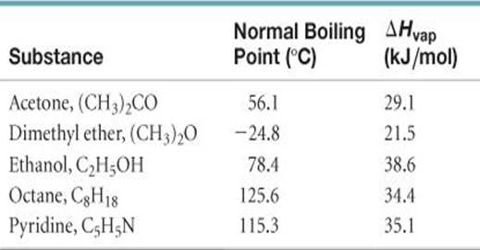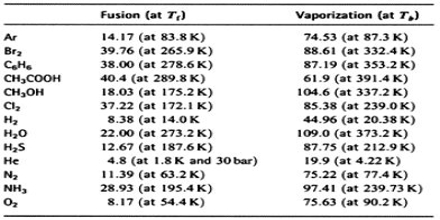# Trouton’s Rule in terms of Enthalpy of Vaporization

Trouton’s Rule in terms of Enthalpy of Vaporization

An empirical rule involving heats of vaporization at the normal boiling point was put forward by F. Trouton. Trouton’s rule states that the heat of vaporization in Joule mol-1 divided by the normal boiling point in Kelvin is approximately 88 for most liquids, i.e.,

∆Hvap/T ≈ 88 J deg-1 mol-1

The entropy of vaporization is defined as the ratio between the enthalpy of vaporization and the boiling temperature.Trouton’s rule is obeyed by a number of liquids as shown in Table.

Data in this Table showed that liquids with wide ranges of boiling points and heats of vaporization give values of the ratio ∆Hvap/T close to 88, although some of the substances show widely different values. Some sort of explanation may be given for such deviation from the Trouton’s rule.

It may be noted that the heat of vaporization divided by the temperature in Kelvin gives the increase in entropy; when a liquid is converted into vapour. For many liquids, then, the increase of entropy during vaporization or the entropy of vaporization is about 88 J deg-1 mol-1.

This means that the increase in disorder mol-1 due to vaporization is the same for a large number of liquids. The liquids which have entropies of vaporization appreciably higher than the average value of 88 J deg-1 mol-1 are those which are known to be associated with the liquid state due to hydrogen bonding. Extra energy would be required to separate the molecules from each other, which makes the entropy of vaporization high. Acetic acid and other carboxyl acids show lower values of ∆Svap. This is to be expected as these acids exist as double molecules even in the vapour state.

A usefulness of the Trouton’s rule is that an approximate value of the boiling point of the liquid may be calculated from this rule if the heat of vaporization is known. The latter quantity may be derived, from the measurement of the vapour pressure of the liquid at two or more temperatures.